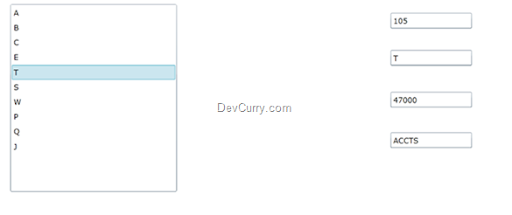# Silverlight: Synchronizing Data between ListBox and Textboxes

 Tweet
In Silverlight applications, a common requirement is to to synchronize data between two controls, like the ListBox and TextBox control bound with the same data source. A ListBox accepts a collection using the ItemsSource property. So when you select any item from the ListBox, the SelectedItem property returns the single object from the collection bound with the ItemsSource. To synchronize data between a ListBox and TextBox (or other elements), we can use the XAML binding facility provided in Silverlight using SelectedItem property of the ListBox. The code is as below:

Step 1: Create a Silverlight application and add the following classes in it:

public class Employee
{
public int EmpNo { get; set; }
public string EmpName { get; set; }
public decimal Salary { get; set; }
public string DeptName { get; set; }
}

public class EmployeeCollection : ObservableCollection<Employee>
{
public EmployeeCollection()
{
Add(new Employee() { EmpNo = 101, EmpName = "A", Salary = 45000, DeptName = "IT" });
Add(new Employee() { EmpNo = 102, EmpName = "B", Salary = 45000, DeptName = "IT" });
Add(new Employee() { EmpNo = 103, EmpName = "C", Salary = 46000, DeptName = "CTD" });
Add(new Employee() { EmpNo = 104, EmpName = "E", Salary = 43000, DeptName = "CTS" });
Add(new Employee() { EmpNo = 105, EmpName = "T", Salary = 47000, DeptName = "ACCTS" });
Add(new Employee() { EmpNo = 106, EmpName = "S", Salary = 495000, DeptName = "SYS" });
Add(new Employee() { EmpNo = 107, EmpName = "W", Salary = 45000, DeptName = "IT" });
Add(new Employee() { EmpNo = 108, EmpName = "P", Salary = 45000, DeptName = "IT" });
Add(new Employee() { EmpNo = 109, EmpName = "Q", Salary = 45000, DeptName = "IT" });
Add(new Employee() { EmpNo = 110, EmpName = "J", Salary = 45000, DeptName = "IT" });
}
}

Step 2: Define the XAML as shown below:

```<UserControl x:Class="SL4_DatabindingDemos.MainPage_ObjectCollection"
xmlns="http://schemas.microsoft.com/winfx/2006/xaml/presentation"
xmlns:x="http://schemas.microsoft.com/winfx/2006/xaml"
xmlns:d="http://schemas.microsoft.com/expression/blend/2008"
xmlns:mc="http://schemas.openxmlformats.org/markup-compatibility/2006"
xmlns:data="clr-namespace:SL4_DatabindingDemos"
mc:Ignorable="d"
d:DesignHeight="332" d:DesignWidth="698">
<UserControl.Resources>
<data:EmployeeCollection x:Key="EmpDs"></data:EmployeeCollection>
<DataTemplate x:Key="EmpNameTemplate">
<TextBlock Text="{Binding EmpName}"></TextBlock>
</DataTemplate>
</UserControl.Resources>
<Grid x:Name="LayoutRoot" Background="White"
DataContext="{Binding Source={StaticResource EmpDs}}">
<Grid.ColumnDefinitions>
<ColumnDefinition Width="314*" />
<ColumnDefinition Width="384*" />
</Grid.ColumnDefinitions>
<ListBox Height="278" HorizontalAlignment="Left" Margin="22,21,0,0"
Name="lstEmp" VerticalAlignment="Top" Width="246"
ItemsSource="{Binding}"
ItemTemplate="{StaticResource EmpNameTemplate}"/>
<Grid Grid.Column="1" Height="269" HorizontalAlignment="Left"
Margin="50,24,0,0" Name="grid1" VerticalAlignment="Top" Width="309"
DataContext="{Binding ElementName=lstEmp,Path=SelectedItem}">
<TextBox Height="23" HorizontalAlignment="Left" Margin="10,10,0,0"
Name="textBox1" Text="{Binding EmpNo}" VerticalAlignment="Top"
Width="120" />
<TextBox Height="23" HorizontalAlignment="Left"
Margin="10,65,0,0" Name="textBox2" Text="{Binding EmpName}"
VerticalAlignment="Top" Width="120" />
<TextBox Height="23" HorizontalAlignment="Left"
Margin="10,127,0,0" Name="textBox3" Text="{Binding Salary}"
VerticalAlignment="Top" Width="120" />
<TextBox Height="23" HorizontalAlignment="Left"
Margin="10,187,0,0" Name="textBox4" Text="{Binding DeptName}"
VerticalAlignment="Top" Width="120" />
</Grid>
</Grid>
</UserControl>
```

The above code defines an instance of the EmployeeCollection class in the XAML using resources. The ListBox is available in Parent Grid (LayoutRoot). This Grid is bound with an instance of the EmployeeCollection class using its DataContext property. The ListBox is set with the Data source using ‘ItemsSource’ property and its ‘ItemTemplate’ is set to the DataTemplate defined in the UserControl resources. The child Grid (Grid1) is bound with the SelectedItem property of the ListBox using DataContext property. When the user selects an EmpName from the ListBox, the Employee object will be created which will be further bound with Grid1’s DataContext property using the below expression
DataContext="{Binding ElementName=lstEmp,Path=SelectedItem}"

This grid contains TextBox children which are bound with the various properties from the Employee class.

Step 3: Run the application and the result will be as shown below: